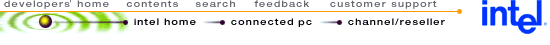## Using MMX™ Instructions to Implement the G.728 Codebook Search

 Disclaimer Information in this document is provided in connection with Intel products. No license, express or implied, by estoppel or otherwise, to any intellectual property rights is granted by this document. Except as provided in Intel's Terms and Conditions of Sale for such products, Intel assumes no liability whatsoever, and Intel disclaims any express or implied warranty, relating to sale and/or use of Intel products including liability or warranties relating to fitness for a particular purpose, merchantability, or infringement of any patent, copyright or other intellectual property right. Intel products are not intended for use in medical, life saving, or life sustaining applications. Intel may make changes to specifications and product descriptions at any time, without notice. Copyright © Intel Corporation (1996). Third-party brands and names are the property of their respective owners.

 1.0. INTRODUCTION 2.1. Algorithm 2.2. MMX™ Code Optimization

## 1.0. INTRODUCTION

The Intel Architecture (IA) media extensions include single-instruction, multiple-data (SIMD) instructions. This document describes an implementation of one of the modules of the G.728 decoder using MMX instructions. G.728 is an algorithm for coding/decoding speech signals at 16 Kbit/s using Low-Delay Code Excited Linear Prediction (LD-CELP). See CCITT recommendation G.728 for a full description of the codec algorithm. The module implemented using MMX instructions is the codebook search (modules 17 and 18) This module receives an input vector and searches through a VQ (Vector Quantization) codebook to identify the closest match.

MMX technology speeds up the codebook search significantly by enabling the efficient parallellization of every operation in the module: multiplication, multiply-accumulate, conditional selection and minimum selection. This document shows how, using MMX technology, parallellism can be achieved even for operations which are inherently serial, such as selection of minimum values.

This document can be used for several purposes:

• For reference when writing an MMX technology optimized version of the G.728 codebook search or a similar codec module.
• For ideas when converting any algorithm to MMX technology.
• For examples of general MMX technology coding and optimization techniques.
• As an example of converting an algorithm from floating point to fixed point and from fixed point to MMX technology.

## 2.0. G.728 CODEBOOK SEARCH

The G.728 codebook search module receives an input vector of length 5. In addition it uses a VQ (Vector Quantization) codebook, which is static, and an array of energy values, which is recomputed for every 4 input vectors. The 10-bit, 1024-entry codebook is decomposed into two smaller codebooks: a 7-bit "shape codebook" containing 128 independent code vectors and a 3-bit "gain codebook" containing 8 scalar values that are symmetric with respect to zero (i.e., one bit for sign, two bits for magnitude). The output of the module is the concatenated index of these two codebooks. The energy array contains the energy of the filtered shape codebook vectors which changes for every four input vectors because the filters change.

### 2.1. Algorithm

This section contains a brief description of the data structures and pseudocode, both for the original (floating-point) algorithm as well as the fixed-point version. Both are described in this document to show an example of converting an algorithm from floating-point to fixed-point; an operation which will often be necessary when designing an MMX technology optimized version for an existing algorithm.

#### ORIGINAL (FLOATING POINT) IMPLEMENTATION

The pseudocode for the floating-point implementation of the algorithm is in Example 1. For a more detailed description of the floating-point implementation, see Sections 3.9 and 5.11 (blocks 17 and 18) of CCITT Recommendation G.728.

Example 1. Floating Point Pseudocode
```
int cb_index (float pn)
{
float d, distm = largest number possible in this representation;
int j, is = 0, ig = 0, idxg, ichan;
float *shape_ptr = &cb_shape, pcor, b0, b1, b2;

for (j=0; j<NCWD; j++)
{
pcor = abs (VDP (shape_ptr[0-4], pn[0-4]));
shape_ptr += 5;

b0 = cb_gain_mid_0*shape_energy[j];
b1 = cb_gain_mid_1*shape_energy[j];
b2 = cb_gain_mid_2*shape_energy[j];
idxg = (if     (pcor < b0) 0
elseif (pcor < b1) 1
elseif (pcor < b2) 2
else               3);

d = gainsq[idxg] * shape_energy[j] - gain2[idxg] * pcor;
if (d < distm)
{
ig = idxg;
is = j;
distm = d;
}
}
shape_ptr = &cb_shape[is];
pcor = VDP (shape_ptr[0-4], pn[0-4]);
if (pcor < 0) ig += 4;
return (concatenation of is and ig);
```

The algorithm loops over the 128 shape vectors in the shape vector codebook. For each shape vector, six steps are performed:

1. Correlation Calculation: performs a correlation (vector dot-product) on the input vector (pn) and the shape vector, both of which have five elements. Then, the absolute value of the correlation result is calculated, yielding the correlation result pcor. The absolute value of pcor is used to simplify the gain index calculation. Because of this, pcor must be recalculated outside the loop to get the correct sign, and the gain value needs to be adjusted accordingly. This is a tradeoff between making the inner loop faster and adding clocks outside the loop. Since the loop is performed 128 times, it results in a net performance gain.
2. Midpoint Calculation: the energy value of the filtered shape vector (which is pre-calculated and kept in the shape_energy array) is multiplied by three constant values to yield three bin midpoints: b0, b1, b2.
3. Gain Index Calculation: the correlation value pcor is compared to the three bin midpoints to find the bin into which it falls (and thus the gain array index idxg.)
4. Gain Index Lookup: a lookup into the gain table is performed with the gain index idxg to find the gain value - actually two table lookups are performed which result in 2*gain and gain^2.
5. Distortion Calculation: a simple calculation (two multiplications and a subtraction) on the gain values, the correlation value, and the energy is performed to find the distortion measurement d. This measure is used to find the shape vector which is the closest match to the input.
6. Minimum Distortion Selection: the distortion measured for the current shape vector is compared to the minimum value found so far. If the distortion of the current vector is smaller than the minimum, then the current vector becomes the new minimum. d and the corresponding gain and shape indexes are saved.

Outside the loop, pcor is recalculated to find the correct sign (since the absolute value was used within the loop), and the gain index is adjusted accordingly. Since the gain table is symmetric with respect to zero this is done by adding 4 to it if pcor is negative. The final result returned is the concatenation of the shape and gain indexes which correspond to the minimum distortion.

#### FIXED-POINT IMPLEMENTATION

The floating-point implementation must be converted to use fixed-point data types before implementation using MMX instructions. This section describes a scalar fixed-point implementation.

#### Fixed-Point Data Formats

One of the main issues when converting an algorithm from floating-point to fixed point is the format to which the data will be converted.

The following values have been converted to 16-bit fixed-point formats: (QX indicates that there are X bits to the right of the fixed radix point. This number is also called the NLS - Number of Left Shifts)

```
cb_shape (shape vector codebook)					(Q11)
shape_energy ](energy array)						(Q5)
pn ](input vector)							(Q7)
cb_gain_mid_0,cb_gain_mid_1,cb_gain_mid_2 (midpoint constants)		(Q13)
gainsq ](table with gain squared)					(Q11)
gain2 ](table with 2*gain)						(Q12)

The following values are in 32-bit fixed-point formats:

pcor (correlation value)						(Q18)
d, distm (distortion and minimum distortion)				(Q16)
b0, b1, b2 (gain bin midpoints)						(Q18)
```

#### Fixed Point Pseudocode

The pseudocode for the floating-point version of the algorithm is in Example 2. For a detailed description of the conversion to fixed-point, see Appendix to TSS Recommendation G.728: Conversion of G.728 to an Implementation With a Fixed Point Arithmetic Device (Section 3.9 in particular).

Example 2. Fixed-Point Pseudocode
```
int cb_index (short pn)
{
int d, distm = (largest number possible in this representation);
int j, is = 0, ig = 0, idxg, ichan;
short *shape_ptr = &cb_shape;
int pcor, b0, b1, b2;

for (j=0; j<NCWD; j++)
{
pcor = abs (VDP (shape_ptr[0-4], pn[0-4])); /* 16 -> 32 bits */
/* NLS for pcor is 18 (11 + 7) */
shape_ptr += 5;

b0 = cb_gain_mid_0*shape_energy[j]; /* 16 -> 32 bits */
b1 = cb_gain_mid_1*shape_energy[j]; /* 16 -> 32 bits */
b2 = cb_gain_mid_2*shape_energy[j]; /* 16 -> 32 bits */
/* NLS for bX are also 18 (13 + 5) */

idxg = (if     (pcor < b0) 0    /* 32 bits comparison */
elseif (pcor < b1) 1    /* 32 bits comparison */
elseif (pcor < b2) 2    /* 32 bits comparison */
else               3);

pcor >>= 14; /* 32 bit shift: NLS of pcor 18 -> 4 */
pcor = clip(pcor, 32767); /* clip pcor(is >0) to 16 bits */
d = gainsq[idxg] * shape_energy[j] - gain2[idxg] * pcor;
/* NLS for d is 11+5=16 and 12+4=16 */

if (d < distm) /* 32 bits comparison */
{
ig = idxg;
is = j;
distm = d;
}
}
shape_ptr = &cb_shape[is];
pcor = VDP (shape_ptr[0-4], pn[0-4]); /* 16 -> 32 bits */
if (pcor < 0) ig += 4; /* 32 bits comparison */
return (concatenation of is and ig);
}
```

Other than the changes in data formats, two more operations have been added in this version: pcor is shifted 14 bits to the left and clipped (with saturation) to 16 bits. This occurs before using pcor in the calculation of d and is needed to adjust the fixed point and precision for this calculation.

### 2.2. MMX™ Code Optimization

This section describes an implementation of the G.728 codebook decoder using MMX instructions.

In these diagrams, each oval represents one MMX instruction. Arrows represent operands and are marked (where the distinction is significant) to show whether the operand is the source or destination, and if it resides in memory.

#### IMPLEMENTATION ALTERNATIVES

The data types in the fixed-point implementation of the codebook search routine range from 16 to 32 bits, so it appears that MMX technology would enable 2X or 4X parallelism. The 16-bit to 32-bit multiply-accumulate operations in the correlation calculation are particularly well-suited to MMX technology. There are two approaches which suggest themselves in paralleling this algorithm for MMX technology. The vector dot-product part of the correlation calculation is implemented using both approaches in order to evaluate which one is more efficient.

#### Alternative I: Parallelism Within One Calculation

This is a standard MMX technology optimized version of a vector dot-product calculation. In this case, the vector is of length 5. To avoid losing performance due to data misalignment each 5-element vector is padded at the end with 3 zero elements. This increases the size of the shape vector codebook by 60% relative to the scalar fixed point version, though it is still 20% smaller than the floating-point version of this array. Figure 1 provides a flow diagram of the vector dot-product portion of the correlation calculation.

Figure 1. Vector Dot-Product (Alternative I)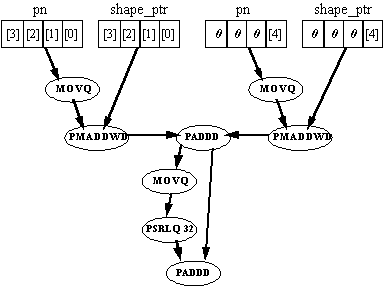Eight instructions are required to perform one vector-dot product calculation. This option is inefficient for two reasons:

1. 3/4 of the parallelism is wasted (3 of every 8 elements are zero).
2. Extra overhead is needed to add the two halves of the accumulator together.

In addition to the fact that this approach is not efficient for the vector dot-product calculation, it does not seem efficient for several other operations in the algorithm which are not inherently parallel.

#### Alternative II: Parallelism Both Within and Between Calculations

This alternative operates on two shape vectors simultaneously, effectively unrolling the inner loop once. There is parallelism both within and between calculations since each pmaddwd instruction performs two multiplications for each of the shape vectors. This approach requires rearranging the shape vector codebook array data, since this array contains only constant values, the rearrangement can be done ahead of time without effecting performance. This type of data rearrangement is typical of efficient MMX technology optimized versions. The new memory order is shown in Figure 2.

Figure 2. New Memory Order of Shape Codebook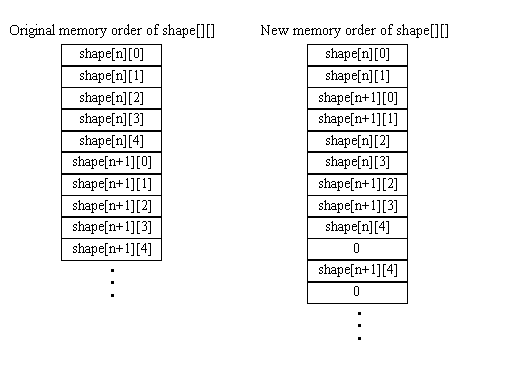The memory space requirement of this array is 20% higher than the scalar fixed-point version but 40% less than that of the floating-point version.

In addition, the input array needs to be rearranged in a similar manner. This can be done quickly with the MMX technology unpack instructions, and is done outside the loop, so the impact on performance is insignificant.

The data flow diagram for the vector dot-product operation in this alternative is shown in Figure 3.

Figure 3. Vector Dot-Product (Alternative II)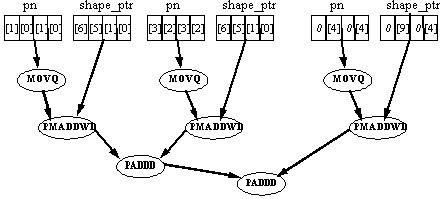Two vector dot-product operations are performed with eight instructions, which is twice as efficient as the previous approach. Also, other sections of the algorithm which are not inherently parallel can be sped up by performing them for two iterations in parallel. Therefore this approach is selected.

#### PROGRAM STRUCTURE

Alternative II, which processes two vectors in parallel, is selected. The loop is unrolled once more, so that two MMX instruction streams can be intermixed, facilitating efficient instruction scheduling (see Section 2.2.4.2). Since each MMX instruction stream operates on two shape vectors, calculations are performed for four shape vectors in each iteration of the loop.

The block diagram in Figure 4 shows one MMX instruction stream processing two shape vectors. The six steps of the inner loop are shown. For each step the number of instructions (within square brackets) and their types is listed. (j refers to the number of the current iteration, cgm0-2 are the cb_gain_mid_X constants.)

Figure 4. Inner Loop Block Diagram (One Instruction Stream)A fractional number of instructions is attributed to the Loop Management and Minimum Distortion Selection sections. This is because they include instructions which only appear in one of the two MMX instruction streams.

#### MMX INSTRUCTION FLOW DIAGRAMS

This section contains detailed instruction flow diagrams for each of the six steps (Correlation, Midpoint, Gain Index Calculation, Gain Index, Distortion, and Minimum Distortion Selection) of the inner loop. Remember that two shape vectors are being processed in parallel in each instruction stream. In addition, two streams are being executed in each iteration of the new inner loop, and some instructions differ between the two streams (these are noted wherever they appear).

#### Correlation Calculation - Step 1

Figure 5. Correlation Calculation Instruction Flow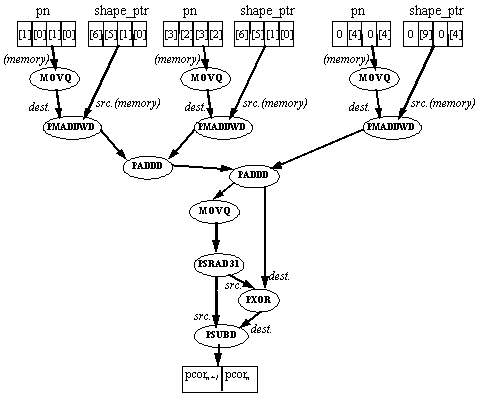The correlation calculation includes two parts: a vector dot-product calculation and an absolute-value calculation.

This absolute-value calculation is performed by a conditional two's-complement operation. The value is copied and shifted arithmetically 31 bits to the left. This creates two doublewords, each of which is 0 if the original doubleword was positive and 0xffffffff (-1) if the original doubleword was negative. The result of this shift is then used to XOR the original value (thus conditionally inverting the bits) and is subtracted from the original value (thus conditionally adding 1). This amounts to conditionally performing a two's complement operation on each doubleword, depending on if it was originally negative - which is the definition of an absolute value calculation.

#### Midpoint Calculation - Step 2

Figure 6. Midpoint Calculation Instruction Flow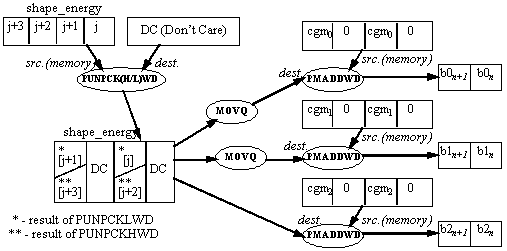In this section of the inner loop the current shape_energy is multiplied by three constants, generating three bin midpoints which are used in the gain index calculation section. Each instruction stream in the MMX code inner loop performs this calculation for two shape_energy values. Taking j as the index to the four elements being processed by the current loop iteration, one instruction stream performs the midpoint calculation on shape_energy[j] and shape_energy[j+1]; the second instruction stream performs this calculation on shape_energy[j+2] and shape_energy[j+3].

Since it is necessary for the results of the multiplication to be in packed doubleword format, it is convenient to use the PMADDWD instruction rather than the PMULLW or PMULHW instructions. The PMADDWD instruction performs four multiplications and two additions. If only two multiplications are needed, the inputs must be padded (at least one of them with zeros).

An unpack operation is performed between the current four elements of shape_energy and a register (the contents of the register are irrelevant). The instruction used is PUNPCKLWD in the first instruction stream, and PUNPCKHWD in the second. The result is a packed word register which contains the appropriate values of shape_energy in its second and fourth word. This result is copied twice, and the three copies are multiplied with the cb_gain_mid_X constants using the PMADDWD instruction. Note that the constants are stored in memory already padded with zeros.

#### Gain Index Calculation - Step 3

Figure 7. Gain Index Calculation Instruction Flow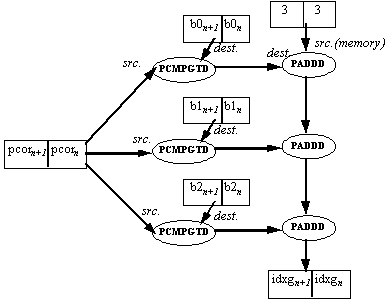In this section of the inner loop PCOR is compared to three bin midpoints, and the gain index is calculated according to the result. (see Pseudocode sequence in Figure 2). Since cannot be made parallel and are slow as well, they are avoided. The 'all 1s' mask generated by a 'true' result in the packed compare instructions is equal to -1 if interpreted as a signed number. Three masks are generated by comparing pcor to the three midpoints using the PCMPGTD instruction. These masks are added to a constant value of 3, to generate a number between 3 and 0 which is the desired gain index. Note that two gain index values are generated in parallel.

#### Gain Index Lookup - Step 4

In this section the gain index is used to perform lookups into two tables: gainsq (the gain values squared) and gain2 (the gain values multiplied by two). A table lookup is an operation which is cannot ordinarily be made parallel using MMX technology, but in this case parallelism is achieved nevertheless. This is possible because the tables are very small - four 16-bit entries each. By creating a two-dimensional array, including all possible combinations of two entries from the gain table, two parallel lookups are possible. If both gain tables are combined into one array, then one lookup yields eight bytes which contain two gain2 and two gainsq values (the gain2 values are stored in negative form, since they are used for subtraction in the Distortion Calculation section). If the tables were much larger this would not be feasible due to the extra memory space requirements and resulting cache problems. The structure of the gain table arrays is in Figure 8, and the instruction flow of the lookup is in Figure 9.

Figure 8. Structure of Gain Table Arrays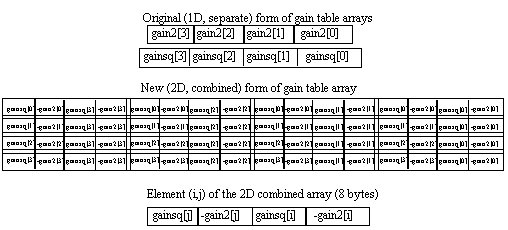Figure 9. Gain Index Lookup Instruction Flow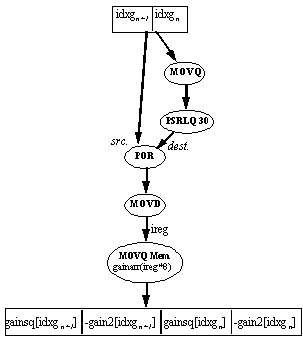The index (4 bits) into the 2D gain table is the concatenation of two 2-bit indexes, each of which is in a separate doubleword (the output of the gain index calculation). To combine them, the gain index register is copied, shifted and ORed. The combined index is moved to an integer register, which is used to access the correct (8 byte) element in the array.

#### Distortion Calculation - Step 5

Figure 10. Distortion Calculation Instruction Flow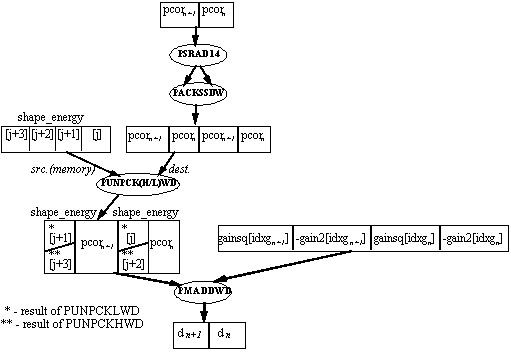In this section the distortion is calculated. Before the actual distortion calculation, pcor must be shifted 14 bits to the right and clipped (with saturation) to 16 bits (see Example 2.). This is accomplished by one packed shift and one pack instruction (it is packed with itself).

The distortion measure d is calculated by the formula:

d = gainsq[idxg] * shape_energy[j] - gain2[idxg] * pcor

This involves two multiplications and a subtraction. If gain2 is stored in negative form, this is two multiplications and an addition, where the inputs have 16-bit precision and the output has 32-bit precision. This is exactly what the PMADDWD instruction does - twice. So one PMADDWD instruction can calculate the distortion for two shape vectors in parallel, if the inputs are formatted correctly. This formatting is performed by one unpack instruction, which interleaves the pcor values with the appropriate shape_energy values. The instruction used is PUNPCKLWD in the first instruction stream, and PUNPCKHWD in the second.

#### Minimum Distortion Selection

Figure 11. Minimum Distortion Selection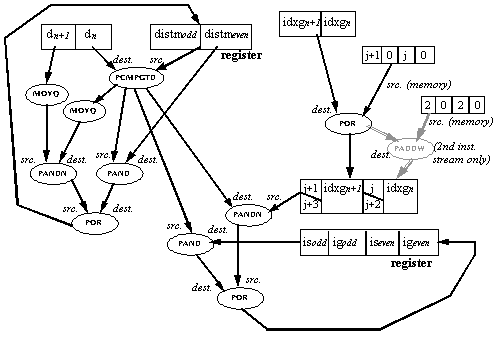In this section the current d is compared to distm (the minimum distortion so far). If d is smaller, then the current codebook shape and gain vectors fit the input vector better then the best fit so far. In this case the best fit is replaced by the current codebook vector, resulting in the following:

• is (shape index of best fit) is replaced by j (current shape codebook index, also number of current iteration)
• ig (gain index of best fit) is replaced by idxg (current gain codebook index)
• distm is replaced by d.

Such a minimum selection operation is inherently serial: the current distm must be found before it can be compared with the next d to find the next distm. However, parallelism can be achieved by using the following method:

Each MMX instruction stream processes two codebook vectors (n and n+1). Packed compare instructions are used for two conditional selection operations in parallel. The best fit found is stored separately for even and odd shape codebook indexes: there is a separate is, ig and distm for odd and even indexes. These are kept separately throughout the inner loop. Only after the inner loop has completed are the two partial minimum values compared to find the actual minimum. Note that the minimum distortion selection of the second instruction stream is dependent on that of the first, so they must be executed in order; this is the only point of dependency between the two instruction streams, which otherwise can be freely intermixed.

The d(n,n+1) and distm(odd,even) values are compared using the PCMPGTD instruction, generating a mask which is used to conditionally select the new distm(odd,even), ig(n,n+1) and is(n,n+1) values. The conditional select operation is done by performing AND and ANDNOT operations with the mask on the new and old values. j/j+1, is(n,n+1) and ig(n,n+1) are in a format which enables doing the conditional select operation for is and ig together. j/j+1 is incremented by 2 for the second instruction stream only (instructions in grey), to become j+2/j+3.

NOTE:

The values is, ig, and distm are kept in 2 registers which are dedicated to holding these values throughout the minimum distortion selection code of both MMX instruction streams. They are loaded from memory before the minimum distortion selection of the first instruction stream and stored after the second. This adds 4 MOVQ instructions per two instruction streams, or an average of 2 MOVQ instructions for each instruction stream.

#### PERFORMANCE OPTIMIZATIONS

This section describes various performance optimizations which are used in the code.

#### Instruction Selection

Throughout the code, an effort was made (by selection of the operand order) to minimize the number of extra movq instructions needed to copy data. In addition, an attempt was made to minimize the number of instructions with memory operands, since these limit the scheduling opportunities.

Instruction length is also worth noting. Instructions with a length exceeding seven bytes were avoided inside the inner loop. The reason for this is that these instructions can reduce pairing, especially if the pairing is otherwise good (as in this code). Note that since MMX instructions have a two-byte opcode, any MMX instruction using a base or index register and a 32-bit displacement or immediate will exceed seven bytes. This is the reason for the use of EDX to store the address of the gain array; to avoid using base + displacement addressing.

#### Register Allocation and Instruction Scheduling

Five out of six sections of the inner loop use four MMX registers or less. This enables the use of two separate sets of MMX registers for the two instruction streams, and freely intermixing instructions (from these five sections) between the streams. In addition, instructions from different sections are also intermixed to enable maximum pairing opportunities. MMX register usage in these sections is as follows:

• The first stream (vectors 0,2,4,...n) uses MMX registers MM0, MM1, MM2 and MM3.
• The second stream (vectors 1,3,5,... n+1) uses MMX registers MM4, MM5, MM6 and MM7.

The minimum distortion selection sections of the two instruction streams have an interdependency, so the instructions of these sections cannot be freely intermixed. MMX register usage in the minimum distortion selection code is as follows:

• The first stream passes values to this section via MM0 and MM1.
• The second stream passes values to this section via MM4 and MM5.
• MM3 and MM7 are used to hold the values of distm(odd,even), ig(n,n+1) and is(n,n+1) throughout the minimum distortion selection code for both instruction streams.
• MM2 and MM6 are used to hold temporary values.

There is some intermixing of instructions between the minimum distortion selection and other sections of code, especially at the beginning and end. In addition, values are preloaded into registers for the correlation calculation of the next iteration (software pipelining).

The result of these techniques is that the code of the inner loop runs with almost perfect pairing (4 unpaired instructions out of 98) and no stalls. The fact that the instruction order is drastically different than the algorithmic order can reduce readability, which is why the code is extensively commented.

#### Data Alignment

The shape and gain codebooks, the energy array and the temps structure (containing the local variables) should be aligned to 8 bytes to avoid costly misalignment penalties. It is less important that the input array be aligned, since it is copied into aligned locations before the start of the inner loop.

The reason that the local variables are passed as a structure and not held on the stack (see code listing) is that they must be aligned to eight bytes to avoid loss of performance. To pass a structure (the caller must ensure alignment) is one solution, another is to add code to the function prologue and epilogue to align the stack itself to eight bytes (see the MMX™ Technology Developer's Manual).

## 3.0. PERFORMANCE

An optimized floating-point version of this routine executes in 4800 clocks. The MMX technology version executes in about 1750 clocks, which is a performance gain of 2.7X. The performance gain is mostly attributable to the MMX instructions which perform operations on multiple data elements and can be paired, unlike the floating-point instructions. These numbers assume that the data is in the L1 cache . This assumption is true when the codebook search is running as part of a G.728 encoder.

This routine is called 1600 times per second when the G.728 encoder is run in real-time. Therefore the 4800 clock floating-point version consumes 5% of the total CPU power of a 150 MHz Pentium processor, while the MMX technology optimized version which takes 1730 clocks reduces this to 2%.

## 4.0. G.728 CODEBOOK SEARCH: CODE LISTING

```
;ProcedureName:
mmx_cb_index

;

;Description:

;   G.728 codebook
search: this is an MMX code implementation of modules 17 and

;   18 of the ITU-T
(fromerly CCITT) G.728 recommendation.

;   Two iterations
of the inner loop have been made parallel, and two of

;   these have been
unrolled and pipelined for a total of four iterations.

;

;C Prototype

;int mmx_cb_index(

;   const short
*mmx_cd_shape,

;   const short
*mmx_energy_result,

;   const short
*pn,

;   short temps[6*4);

;

;NOTE:

; This is a fixed-point
version of the algorithm. In comments, the format

; of various variables/tables/constants
will be described as xQy where

; x is the number
of bits (16 or 32) and y is the number of bits to the

; right of the implicit
radix point. (Example: 16Q5). Where there is an

; MMX code-type
variable which includes 4 16-bit or 2 32-bit values, the format

; will be described
as: 2x32Q5, or 4x16Q7, etc.

;NOTE:

refers to 'Don't Care' values.

;

;Internal Register
Usage:

; IA: (Note that
it is assumed that the calling program does not need

;      eax,ecx,
or edx to be preserved - this is true for C programs compiled

;      with Microsoft
Visual C++, for example).

;   eax - general
use, and return value

;   ebx - pointer
into int16_shape_energy: equal to int16_shape_energy + j*8

;         it is
also used for checking loop termination.

;   ecx - pointer
(s_p) into shape codebook array (mmx_cb_shape)

;   edx - pointer
to start of gain array (gainarr) (this is to use base+index

when accessing this array instead of displacement+index.

;         This reduces
the size below 8 bytes, and so facilitates pairing).

;   edi - pointer
to temporary data of 6 quadwords: pn01, pn23, pn4z, distm,

;         ig_is,
and j_jp1

;   esi - pointer
to to end of shape energy for loop termination

; MMx:

;

;Inputs:   Uses
C language stack frame

; Globals:

;

; Input Parameters:

;   mmx_cb_shape:PTR
WORD - ptr to start of codebook.

;      NOTE: The
elements in the codebook have been rearranged to facilitate

;            speeding
up the algorithm. When comments refer to non-consecutive

;            indices
in the shape array for example s_p[0,1,5,6], this refers

;            to
the elements in the original, non-rearranged codebook: in

;            memory,
these elements actually appear in this order.

;

;   int16_shape_energy:
PTR WORD - ptr to start of energy array.

;   pn:PTR WORD
- ptr to start input vector (5 x 16bit vector)

;   temps: pointer
to 6 aligned qwords used for:

;   pn01:   QWORD
- duplicated and aligned copy of pn and pn

;   pn23:   QWORD
- duplicated and aligned copy of pn and pn

;   pn4z:   QWORD
- duplicated and aligned copy of pn (padded with 0s)

;   distm:   QWORD
- distm(even),distm(odd) (2x32Q16) (init to max 32bit)

;   ig_is   QWORD
- ig(even),is(even),ig(odd),is(odd) (4x16)

;   j_jp1   QWORD
- 0, j, 0, j+1 (4x16) (initialised to 0,-2,0,-1)

;

;Locals: (in the
data segment, not the stack)

; Constants:

;   cgm0:   4 x
SWORD - cb_gain_mid_0, duplicated and padded (w. zeros)

;   cgm1:   4 x
SWORD - cb_gain_mid_1, duplicated and padded (w. zeros)

;   cgm2:   4 x
SWORD - cb_gain_mid_2, duplicated and padded (w. zeros)

;   gainarr:64 x
SWORD - combination and squaring of gain2 & gainsq arrays

;   val01:   4 x
WORD - 0,-4,0,-3 (for initialising j/j+1)

;   inc04   4 x
WORD - 0,4,0,4 (for incrementing j/j+1)

;   inc02:   4 x
WORD - 0,2,0,2 (for converting j/j+1 into j+2/j+3)

;   val33:   2 x
DWORD -  3,3

;   max32:   2 x
SDWORD - maximum signed 32-bit value, twice (init. for distm)

;   val12_0:4 x
SWORD - 12,0,0,0

;   val32:   QWORD
- 32

WORD - 0FFFFh, 0, 0FFFFh, 0 (mask out high word in each dword)

;

;Return Value:

;   16-bit integer,
in eax - the concatenated gain and codebook indexes for

;   the best fit.

;****************************************************************************

INCLUDE iammx.inc
;IA-MMx emulator macros

.486P

.model FLAT

_DATA SEGMENT PARA
PUBLIC USE32 'DATA'

pn01    equ   [edi]
;pn,pn,pn,pn

pn23    equ   [edi+8]
;pn,pn,pn,pn

pn4z    equ   [edi+16]
;pn,0,pn,0

distm   equ   [edi+24]
;distm(even,odd)

ig_is   equ   [edi+32]
;ig,is(even,odd)

j_jp1   equ   [edi+40]
;0,j,0,j+1

cgm0    SWORD

cgm1    SWORD

cgm2    SWORD

gainarr SWORD
-4224,545,-4224,545,

-7392,1668,-4224,545,

-12936,5107,-4224,545,

-22638,15640,-4224,545,

-4224,545,-7392,1668,

-7392,1668,-7392,1668,

-12936,5107,-7392,1668,

-22638,15640,-7392,1668

SWORD
-4224,545,-12936,5107,

-7392,1668,-12936,5107,

-12936,5107,-12936,5107,

-22638,15640,-12936,5107,

-4224,545,-22638,15640,

-7392,1668,-22638,15640,

-12936,5107,-22638,15640,

-22638,15640,-22638,15640

val01   SWORD
0,-4,0,-3

inc04   SWORD
0,4,0,4

inc02   SWORD
0,2,0,2

val33   DWORD
3,3

max32   SDWORD
7FFFFFFFh,7FFFFFFFh      ;maximum 32-bit signed value x 2

val12_0 SWORD
12,0,0,0

val32   QWORD
32

;mask out high word in each dword

_DATA ENDS

_TEXT SEGMENT PARA
PUBLIC USE32 'CODE'

mmx_cb_index PROC
NEAR C PUBLIC USES EBX EDI ESI,

mmx_cb_shape:PTR
WORD, int16_shape_energy: PTR WORD, pn: DWORD, temps: PTR WORD

;Initialisation

;--------------

;initialise pointer
into mmx_cb_shape (to last 4 vectors in array)

;it will be incremented
by 48 at the end of every 4 iterations.

mov         ecx,
mmx_cb_shape

;initialise pointer
into int16_shape_energy:  it is equal to

;int16_shape_energy
+ j*8. t is also used for checking loop termination.

mov         ebx,
int16_shape_energy

;initialise pointer
to start of gain array (gainarr) (this is to use

when accessing this array instead of

;displacement+index.
this reduces the size below 8 bytes, and so

;facilitates
pairing).

mov         edx,
OFFSET gainarr

;initialize pointer
to start of temporary data provided by caller

mov         edi,
temps

;initialize pointer
to end of int16_shape_energy, for loop termination.

lea         esi,
[ebx + 256]

;initialise distm(even,odd)
to maximum integer values

movq        mm5,
max32

movq        DWORD
PTR distm, mm5

;initialise j/j+1
to 0,-4,0,-3

movq        mm7,
DWORD PTR val01

movq        DWORD
PTR j_jp1, mm7

;initialise
pn01 with pn,pn,pn,pn

mm7 registers to prepare for start of loop

mov         eax,
pn

movq        mm3,
[eax]            ;mm3 contains pn[0-3]

movq        mm2,
mm3

punpckldq   mm3,
mm3              ;mm3 contains pn,pn,pn,pn

movq        DWORD
PTR pn01, mm3   ;move to pn01

movq        mm7,
mm3              ;now both mm3, mm7 are initialized

;initialise pn23
with pn,pn,pn,pn

mm6 registers to prepare for start of loop

punpckhdq   mm2,
mm2              ;mm2 contains pn,pn,pn,pn

movq        DWORD
PTR pn23, mm2   ;move to pn23

movq        mm6,
mm2              ;now both mm2, mm6 are initialized

;initialise pn4z
with pn,0,pn,0

mm5 registers to prepare for start of loop

movq        mm1,
8[eax]           ;mm1 contains pn,0,DC,DC

punpckldq   mm1,
mm1              ;mm1 contains pn,0,pn,0

movq        DWORD
PTR pn4z, mm1   ;move to pn4z

movq        mm5,
mm1              ;now both mm1, mm5 are initialized

nop
;This nop is to avoid a MASM bug

;Align start of
loop to 16 bytes

align 16

nop
;This nop is to avoid a MASM bug

START_LOOP:

show for each instruction: to which part of the

;algorithm it belongs,
and to which pair of iterations - (j,j+1) or

;(j+2,j+3). This
is to facilitate readability after software pipelining

;is performed (which
will mix instructions from different sections

;and iterations)

;NOTE: here spacing
between instructions indicates expected pairing on Pentium Pro.

;Start of loop

;-------------

;In this section
5 sections of both iteration pairs (j,j+1) and

;(j+2,j+3) are

;1. correlation
(corr) - do VDP between pn and shape_ptr (s_p), abs.

;   Note that
out of the instruction which load pn[] into registers,

;   some appear
here and some appear at the end of the loop (and before

;   the start
of the loop).

;   NOTE - in
this section comments such as s_p[0,1,5,6] refer to the

;          order
of the elements in the original, non-rearranged codebook:

;          the
array which is accessed by s_p is arranged so that these

;          elements
appear in this order in memory.

;2. midpoint
calculation (mid) - unpack low 2 elements (out of

;   current
4) of int16_shape_energy with an (uninitialised) register,

;   then multiply
w. cgm0,1,2 to produce b0,1,2.

;3. gain index
calculation (gidx) - compare pcor(j,j+1)/(j+2,j+3)

;   to bX(j,j+1)/(j+2,j+3)
then add results (-1 if bX > pcor, 0 else)

;   to 3.

;4. gain index
lookup (glook) - copy/shift logic right(by 30) & OR

;   idxg w. itself
to combine (j,j+1)/(j+2,j+3) indices into one index,

;   then do lookup
w. this combined index into a combined -gain2,

;   gainsq table.

;5. distortion
calculation (dcalc)- shift pcor right by 14, and pack it

;   w. itself
(thus saturating it to 16 bits and copying it). Then do

;   unpack low
with shape_energy, and pmadd w. gain vector (mm2)

;   (d=int16_shape_energy*gainsq
- pcor*gain2)

; Every line
of code is commented as to which section and iteration

; pair it belongs
to.

;Note also that
instructions related to loop management (loop_mgmt)

;are scattered
throughout the code.

;The two iteration
pairs can be freely made parallel since (in these

;sections) they
use 2 distinct groups of 4 MMX registers each:

;(j,j+1) uses
mm0,mm1,mm2,mm3 and (j+2,j+3) uses mm4,mm5,mm6,mm7.

[ecx]            ;corr(j,j+1): pn01*s_p[0,1,5,6]

movq        mm6,
mm2              ;corr(j+2,j+3): load pn23 into reg.

8[ecx]           ;corr(j,j+1): pn23*s_p[2,3,7,8]

movq        mm5,
mm1              ;corr(j+2,j+3): load pn4z into reg.

24[ecx]          ;corr(j+2,j+3): pn01*s_p[12,13,17,18]

32[ecx]          ;corr(j+2,j+3): pn23*s_p[14,15,19,20]

16[ecx]          ;corr(j,j+1): pn4z*s_p,0,,0

mm3              ;corr(j,j+1): 1st accumulate

40[ecx]          ;corr(j+2,j+3): pn4z*s_p,0,,0

movq        mm0,
DWORD PTR j_jp1  ;loop_mgmt: load j_jp1 for incrementing

mm7              ;corr(j+2,j+3): 1st accumulate

DWORD PTR inc04  ;loop_mgmt: inc. j/j+1 by 0,4,0,4

mm2              ;corr(j,j+1): 2nd acc, VDP in mm1

movq        mm3,
mm1              ;corr(j,j+1): start abs (copy)

mm6              ;corr(j+2,j+3): 2nd acc, VDP in mm5

movq        DWORD
PTR j_jp1, mm0  ;loop_mgmt: store incremented j/j+1

31               ;corr(j,j+1): shift the copy

punpcklwd   mm0,
[ebx]            ;mid(j,j+1): s_e[j],[j+1]

pxor        mm1,
mm3              ;corr(j,j+1): xor w. shifted

movq        mm2,
mm0              ;mid(j,j+1): copy

movq        mm7,
mm5              ;corr(j+2,j+3): start abs (copy)

DWORD PTR cgm1   ;mid(j,j+1): b1(j,j+1) in mm2

psubd       mm1,
mm3              ;corr(j,j+1): sub, abs in mm1

;here the correlation
result pcor(j,j+1) is in mm1

punpckhwd   mm4,
[ebx]            ;mid(j+2,j+3): s_e[j+2],[j+3]

movq        mm3,
mm0              ;mid(j,j+1): copy

DWORD PTR cgm0   ;mid(j,j+1): b0(j,j+1) in mm3

31               ;corr(j+2,j+3): shift the copy

DWORD PTR cgm2   ;mid(j,j+1): b2(j,j+1) in mm0

pxor        mm5,
mm7              ;corr(j+2,j+3): xor w. shifted

psubd       mm5,
mm7              ;corr(j+2,j+3): sub, abs in mm5

;here the correlation
result pcor(j+2,j+3) is in mm5

movq        mm7,
mm4              ;mid(j+2,j+3): copy

DWORD PTR cgm0   ;mid(j+2,j+3): b0(j+2,j+3) in mm7

pcmpgtd     mm2,
mm1              ;gidx(j,j+1): compare pcor to b1

movq        mm6,
mm4              ;mid(j+2,j+3): copy

pcmpgtd     mm3,
mm1              ;gidx(j,j+1): compare pcor to b0

val33            ;gidx(j,j+1): add b0 cmp results

pcmpgtd     mm0,
mm1              ;gidx(j,j+1): compare pcor to b2

DWORD PTR cgm1   ;mid(j+2,j+3): b1(j+2,j+3) in mm6

mm3              ;gidx(j,j+1): add b1 cmp results

DWORD PTR cgm2    ;mid(j+2,j+3): b1(j+2,j+3) in mm4

mm2               ;gidx(j,j+1): add b2 cmp results

;here idxg(j,j+1)
is in mm0.

pcmpgtd     mm7,
mm5              ;gidx(j+2,j+3): compare pcor to b0

movq        mm2,
mm0              ;glook(j,j+1): copy idxg

pcmpgtd     mm6,
mm5              ;gidx(j+2,j+3): compare pcor to b1

psrlq       mm2,
30               ;glook(j,j+1): shift copy right

pcmpgtd     mm4,
mm5              ;gidx(j+2,j+3): compare pcor to b2

por         mm2,
mm0              ;glook(j,j+1): or idxg w. copy

val33            ;gidx(j+2,j+3): add b0 cmp results

14               ;dcalc(j,j+1): pcor>>= 14: pcor>>=
14

movd        eax,
mm2              ;glook(j,j+1): mov to index reg.

packssdw    mm1,
mm1              ;dcalc(j,j+1): pack pcor, sat to 16

;here mm1 contains
pcor(j),pcor(j+1),pcor(j),pcor(j+1)

punpcklwd   mm1,
[ebx]            ;dcalc(j,j+1):pcor,s_e[j,j+1]

;here mm1 contains:

;pcor(j),int16_shape_energy[j],pcor(j+1),int16_shape_energy[j+1]

mm7              ;gidx(j+2,j+3): add b1 cmp results

movq        mm2,
DWORD PTR [edx+eax*8];glook(j,j+1): lookup w. index

;here mm2 contains:

;-gain2[idxg(j)],gainsq[idxg(j)],-gain2[idxg(j+1)],gainsq[idxg(j+1)]

mm6              ;gidx(j+2,j+3): add b2 cmp results

;here idxg(j+2,j+3)
is in mm4.

mm2              ;dcalc(j,j+1): calc d(j,j+1)

;here mm1 contains
d(j),d(j+1)

movq        mm6,
mm4              ;glook(j+2,j+3): copy idxg

;Here the 5 sections
of the iteration pair (j,j+1) have completed

;execution. We
parallelise the remaining sections (dcalc and part of

;glook) of (j+2,j+3)
with the start of the 'mind' module of (j,j+1).

;minimum distortion
selection (mind): do conditional mov's between

;new and old
dist, ig/is.

;distm(even,odd)
is compared w. d(j,j+1)and distm and ig/is are

;updated accordingly.

por         mm0,
DWORD PTR j_jp1  ;mind(j,j+1): OR j/j+1 w. idxg

;here mm0 contains
idxg(j),j,idxg(j+1),j+1]

psrlq       mm6,
30               ;glook(j+2,j+3): shift copy right

por         mm6,
mm4              ;glook(j+2,j+3): or idxg w. copy

14               ;dcalc(j+2,j+3): pcor>>= 14:

movq        mm3,
DWORD PTR distm  ;mind(j,j+1): load mm3 w. distm

movq        mm2,
mm1              ;mind(j,j+1): copy d

movd        eax,
mm6              ;glook(j+2,j+3): mov to index reg.

packssdw    mm5,
mm5              ;dcalc(j+2,j+3): pack pcor, sat to 16

;here mm5 contains
pcor(j+2),pcor(j+3),pcor(j+2),pcor(j+3)

punpckhwd   mm5,
[ebx]            ;dcalc(j+2,j+3):w. s_e[j+2,j+3]

;here mm5 contains:

;pcor(j+2),int16_shape_energy[j+2],pcor(j+3),int16_shape_energy[j+3]

pcmpgtd     mm1,
mm3              ;mind(j,j+1): cmp distm to d

;here mm1 contains
a mask (1's = d>distm) (2x32)

movq        mm6,
DWORD PTR [edx+eax*8];glook(j+2,j+3): lookup w. index

;here mm6 contains:

;-gain2[idxg(j+2)],gainsq[idxg(j+2)],-gain2[idxg(j+3)],gainsq[idxg(j+3)]

pand        mm3,
mm1              ;mind(j,j+1): AND distm w. mask

mm6              ;dcalc(j+2,j+3): calc d(j+2,j+3)

;here mm5 contains
d(j+2),d(j+3)

movq        mm6,
mm1              ;mind(j,j+1)

;In this section
we just have mind(j,j+1,(j+2,j+3) made parallel with

;some loop management(loop_mgmt)
and some instructions from correlation

;calculation
(corr).

movq        mm7,
DWORD PTR ig_is  ;mind(j,j+1): load mm7 w. ig_is

pandn       mm6,
mm2              ;mind(j,j+1): AND d w. reverse mask

por         mm3,
mm6              ;mind(j,j+1): OR masked d, distm

;conditional
move on distm done - now do cond. move on is, ig

pand        mm7,
mm1              ;mind(j,j+1): AND ig,is w. mask

por         mm4,
DWORD PTR j_jp1  ;mind(j+2,j+3): OR j/j+1 w. idxg

pandn       mm1,
mm0              ;mind(j,j+1): AND idxg,j w. inv mask

DWORD PTR inc02  ;add 0,2,0,2 to make j+2/j+3

;here mm4 contains
idxg(j+2),j+2,idxg(j+3),j+3]

por         mm7,
mm1              ;mind(j,j+1): OR masked idxg/j, ig/is

movq        mm2,
mm5              ;mind(j+2,j+3): copy d

pcmpgtd     mm5,
mm3              ;mind(j+2,j+3): cmp distm to d

;here mm5 contains
a mask (1's = d>distm) (2x32)

movq        mm6,
mm5              ;mind(j+2,j+3)

pand        mm3,
mm5              ;mind(j+2,j+3): AND distm w. mask

pandn       mm6,
mm2              ;mind(j+2,j+3): AND d w. inv mask

pand        mm7,
mm5              ;mind(j+2,j+3): AND ig,is w. mask

movq        mm2,
DWORD PTR pn23   ;corr(j,j+1): load pn23 into reg.

pandn       mm5,
mm4              ;mind(j+2,j+3): idxg,j AND inv mask

movq        mm1,
DWORD PTR pn4z   ;corr(j,j+1): load pn4z into reg.

por         mm3,
mm6              ;mind(j+2,j+3): OR masked d, distm

48               ;loop_mgmt: increment s_p by 48

8                ;loop_mgmt: increment pointer by 8

movq        DWORD
PTR distm, mm3  ;mind(j+2,j+3): store mm3 in distm

por         mm7,
mm5              ;mind(j+2,j+3): merge idxg/j, ig/is

movq        mm3,
DWORD PTR pn01   ;corr(j,j+1): load pn01 into reg.

movq        DWORD
PTR ig_is, mm7  ;mind(j+2,j+3): store mm7 in ig_is

movq        mm7,
mm3              ;corr(j+2,j+3): load pn01 into reg.

cmp         ebx,
esi              ;loop_mgmt

jb          START_LOOP
;loop_mgmt: branch

;After loop - postprocess
results

;--------------------------------

;In this section
we first find the minimum out of distm(even)/distm(odd), and

;select is and ig
accordingly.

;Then pcor is recalculated
and 4 added to ig if it is negative.

;format is,
ig so that _ig_ is in 3 LSBits, and _is_ is in 7 bits above.

;(currently ig
is in bits 0-15. and is in bits 16-31).

movq        mm6,
DWORD PTR ig_is

movq        mm3,
mm6

psrld       mm6,
13

por         mm6,
mm3

pand        mm6,

;select is,
ig according to minimum distm.

movq        mm5,
DWORD PTR distm

movd        eax,
mm5              ;distm(even) -> eax

psrlq       mm5,
32

movd        ebx,
mm5              ;distm(odd) -> ebx

pxor        mm7,
mm7              ;initialise even/odd indicator

cmp         eax,
ebx

jle         MIN_EVEN
;if distm(even)<=distm(odd), jmp

movq        mm7,
DWORD PTR val32  ;mm7 is 32 if distm(odd) is min, 0 else

MIN_EVEN:

psrlq       mm6,
mm7              ;shift ig/is(min) into low 32 bits

movq        mm4,
mm6              ;ig/is of minimum distm -> mm4

psrld       mm6,
4                ;now LSW of mm6 has is/2

pmullw      mm6,
DWORD PTR val12_0 ;multiplied by 12 for index into codebook

movd        esi,
mm6

;recalculate
VDP

mov         ecx,
mmx_cb_shape     ;point ecx back to begining of codebook

movq        mm0,
DWORD PTR pn01

movq        mm1,
DWORD PTR pn23

movq        mm2,
DWORD PTR pn4z

[ecx+esi*2]

[ecx+esi*2+8]

mm0

[ecx+esi*2+16]

mm1

;here recalculated
pcor is in mm2 (either the high or low DWord)

psrlq       mm2,
mm7              ;shift pcor into low 32 bits

;here recalculated
pcor is in low DWord of mm2 (high dword may have garbage)

;if result negative,

psrld       mm2,
31

pslld       mm2,
2

por         mm4,
mm2

movd        eax,
mm4              ;return value

emms
;clear MM state

ret   0

mmx_cb_index ENDP

_TEXT   ENDS

END

@```Legal Stuff © 1997 Intel Corporation